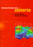© CAMBRIDGE UNIVERSITY PRESS 1999

1.6.2 Cosmic Inflation: Introduction

The basic idea of inflation is that before the universe entered the present adiabatically expanding Friedmann era, it underwent a period of de Sitter exponential expansion of the scale factor, termed inflation (Guth 1981). Actually, inflation is never precisely de Sitter, and any superluminal (faster-than-light) expansion is now called inflation. Inflation was originally invented to solve the problem of too many GUT monopoles, which, as mentioned in the previous section, would otherwise be disastrous for cosmology.

The de Sitter cosmology corresponds to the solution of Friedmann's equation in an empty universe (i.e., with= 0) with vanishing curvature (k = 0) and positive cosmological constant (> 0). The solution is a = a0 eHt, with constant Hubble parameter H = (/3)1/2. There are analogous solutions for k = +1 and k = -1 with acosh Ht and asinh Ht respectively. The scale factor expands exponentially because the positive cosmological constant corresponds effectively to a negative pressure. de Sitter space is discussed in textbooks on general relativity (for example, Rindler 1977, Hawking & Ellis 1973) mainly for its geometrical interest. Until cosmological inflation was considered, the chief significance of the de Sitter solution in cosmology was that it is a limit to which all indefinitely expanding models with> 0 must tend, since as a ->, the cosmological constant term ultimately dominates the right hand side of the Friedmann equation.

As Guth (1981) emphasized, the de Sitter solution might also have been important in the very early universe because the vacuum energy that plays such an important role in spontaneously broken gauge theories also acts as an effective cosmological constant. A period of de Sitter inflation preceding ordinary radiation-dominated Friedmann expansion could explain several features of the observed universe that otherwise appear to require very special initial conditions: the horizon, flatness/age, monopole, and structure formation problems. (See Table 1.6.)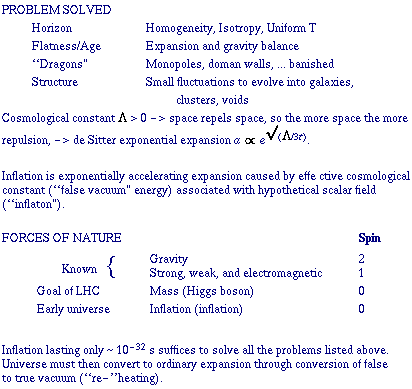Let us illustrate how inflation can help with the horizon problem. At recombination (p+ + e- -> H), which occurs at a / a010-3, the mass encompassed by the horizon was Mh1018 M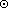, compared to Mh,01022 Mtoday. Equivalently, the angular size today of the causally connected regions at recombination is only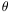~ 3°. Yet the fluctuation in temperature of the cosmic background radiation from different regions is very small:T/T ~ 10-5. How could regions far out of causal contact have come to temperatures that are so precisely equal? This is the ``horizon problem''. With inflation, it is no problem because the entire observable universe initially lay inside a single causally connected region that subsequently inflated to a gigantic scale. Similarly, inflation exponentially dilutes any preceeding density of monopoles or other unwanted relics (a modern version of the ``dragons'' that decorated the unexplored borders of old maps).

In the first inflationary models, the dynamics of the very early universe was typically controlled by the self-energy of the Higgs field associated with the breaking of a Grand Unified Theory (GUT) into the standard 3-2-1 model: GUT -> SU(3)color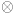[SU(2)U(1)]electroweak. This occurs when the cosmological temperature drops to the unification scale TGUT ~ 1014 GeV at about 10-35 s after the Big Bang. Guth (1981) initially considered a scheme in which inflation occurs while the universe is trapped in an unstable state (with the GUT unbroken) on the wrong side of a maximum in the Higgs potential. This turns out not to work: the transition from a de Sitter to a Friedmann universe never finishes (Guth & Weinberg 1981). The solution in the ``new inflation'' scheme (Linde 1982; Albrecht and Steinhardt 1982) is for inflation to occur after barrier penetration (if any). It is necessary that the potential of the scalar field controlling inflation (``inflaton'') be nearly flat (i.e., decrease very slowly with increasing inflaton field) for the inflationary period to last long enough. This nearly flat part of the potential must then be followed by a very steep minimum, in order that the energy contained in the Higgs potential be rapidly shared with the other degrees of freedom (``reheating''). A more general approach, ``chaotic'' inflation, has been worked out by Linde (1983, 1990) and others; this works for a wide range of inflationary potentials, including simple power laws such as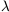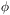4. However, for the amplitude of the fluctuations to be small enough for consistency with observations, it is necessary that the inflaton self-coupling be very small, for example~ 10-14 for the4 model. This requirement prevents a Higgs field from being the inflaton, since Higgs fields by definition have gauge couplings to the gauge field (which are expected to be of order unity), and these would generate self-couplings of similar magnitude even if none were present. Both the Higgs and inflaton are hypothetical fundamental (or possibly composite) scalar fields (see Table 1.6).

It turns out to be necessary to inflate by a factor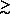e66 in order to solve the flatness problem, i.e., that0 ~ 1. (With H-1 ~ 10-34 s during the de Sitter phase, this implies that the inflationary period needs to last for only a relatively small time10-32 s.) The ``flatness problem'' is essentially the question why the universe did not become curvature dominated long ago. Neglecting the cosmological constant on the assumption that it is unimportant after the inflationary epoch, the Friedmann equation can be written(1.6)

where the first term on the right hand side is the contribution of the energy density in relativistic particles and g (T) is the effective number of degrees of freedom. The second term on the right hand side is the curvature term. Since aTconstant for adiabatic expansion, it is clear that as the temperature T drops, the curvature term becomes increasingly important. The quantity Kk / (aT)2 is a dimensionless measure of the curvature. Today, |K| = |- 1| H02 / T022 x 10-58. Unless the curvature exactly vanishes, the most ``natural'' value for K is perhaps K ~ 1. Since inflation increases a by a tremendous factor eHat essentially constant T (after reheating), it increases aT by the same tremendous factor and thereby decreases the curvature by that factor squared. Setting e-2H2 x 10-58 gives the needed amount of inflation: H66. This much inflation turns out to be enough to take care of the other cosmological problems mentioned above as well.

Of course, this is only the minimum amount of inflation needed; the actual inflation might have been much greater. Indeed it is frequently argued that since the amount of inflation is a tremendously sensitive function of e.g., the initial value of the inflaton field, it is extremely likely that there was much more inflation than the minimum necessary to account for the fact that0 is of order unity. It then follows that the curvature constant is probably vanishingly small after inflation, which implies (in the absence of a cosmological constant today) that0 = 1 to a very high degree of accuracy. A way of evading this that has recently been worked out is discussed below.